/

### Function Description

Returns a vector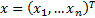(and three further values indicating the total asset weight, the return and the risk of the portfolio in that order) that maximises the following investor utility function subject to lower bound constraints of the form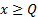and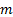further (linear) constraints of the formand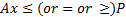.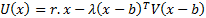Here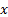are the portfolio weights (so typically we impose at least the following constraint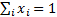),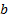is the benchmark (or ‘minimum risk’ portfolio),is a vector of assumed returns on each asset and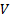is the covariance matrix (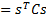, whereis the vector of risks on each asset class, here assumed to be characterised by their volatilities, as this approach is merely a mean-variance one, and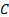their correlation matrix).

Constraints are coded -1 for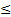, 0 for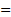and +1 for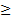. For example, if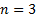,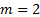and the additional linear constraints are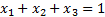and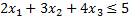then: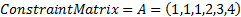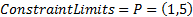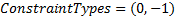Contents | Prev | Next